# Catch up on the latest AI articles# What Is An Appropriate Label Representation In Image Classification?

3 main points
✔️ Improved image classification task performance by using audio data as labels
✔️ Experiments and comparisons using various label representations

✔️
Demonstrate that high-dimensional and high-entropy label representations improve robustness and data efficiency

written by Boyuan ChenYu LiSunand RaghupathiHod Lipson
(Submitted on 6 Apr 2021)
Comments: Accepted to  International Conference on Learning Representations (ICLR 2021).

Subjects: Machine Learning (cs.LG); Artificial Intelligence (cs.AI); Computer Vision and Pattern Recognition (cs.CV)code：## First of all

In the image classification task, class prediction is performed for input images. In this case, how does the representation and format of the label, which is the teacher data, affect the performance of the model?

In the paper presented in this article, we used a variety of labels, including audio data, and performed various tests on how to label representation affects model performance to provide an answer to the previous question.

## Experiment

### Experiment setup

Data set

Using the CIFAR-10/100 dataset, we experiment with using various variants as label representations in image classification.

Voice label

The following procedure is used to generate voice labels.

• English speech is generated from the text corresponding to the category by the TTS (text-to-Speech) system. In this case, all speech labels are generated with the same parameters and API.
• Each audio file is saved in WAVE format with 16-bit pulse code modulation encoding, and the silence at both ends is trimmed.
• It converts to a Mel spectrogram with a sampling rate of 22,050 Hz, a frequency of 64 Mel, and a hop length of 256.
• We turn the spectrogram into an N (twice the input image dimension) × N matrix with values from -80 to 0 and use this as the label.

This voice label corresponds to each class, one for each class.

More labels

In order to investigate what properties of the labels affect which, we will conduct experiments using the various labels shown below.

• Shuffled Speech: Divides the speech spectrogram image into 64 parts along the time dimension and reorders them. This process preserves the entropy and dimensionality of the original speech labels.
• constant-matrix: uses a dimension where all elements have the same value (zero entropy).
• Gaussian-composition: Combines 10 Gaussians uniformly sampled in position and orientation.
• random/uniform-matrix: Generates a matrix with randomly sampled elements from a uniform distribution. It also uses variants with lower dimensionality to investigate the importance of dimensionality.
• BERT embedding: uses the embedding of the last hidden layer of the pre-trained BERT model.
• GloVe embedding: Similar to the BERT model, we use word embedding from a pre-trained GloVe model.

An example of label visualization including the aforementioned audio labels is shown below.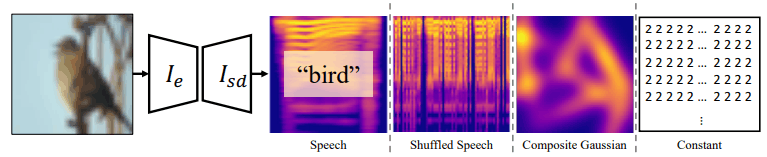・Model

We use the following three CNNs in our model.

The categorization model (baseline setting) consists of an image encoder $I_e$ and a category (text) decoder $I_{td}$, where $I_e$ is the CNN backbone described earlier and $I_{td}$ is the full coupling layer.

In the model that uses high-dimensional labels, the image encoder remains the same and the label decoder $I_{ld}$ is used instead. The $I_{ld}$ consists of one dense layer and several transposes convolutional layers.

## Training and Evaluation

The categorization model is trained by cross-entropy loss, and when using high-dimensional labels, the model is trained to minimize the following equation (Huber loss is used)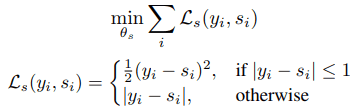Categorization models, like general classification tasks, are considered correct if the class predicted with the highest probability is the same as the target class. High-dimensional labels are measured in two different forms.

• Select the ground-truth label that is closest to the output of the model (NN: nearest neighbor).
• A prediction is considered correct if the Huber loss (smooth L1 loss) with the true label is below a certain threshold (3.5 in the experiment).

## Experimental results

The classification accuracies for all the aforementioned labels are as follows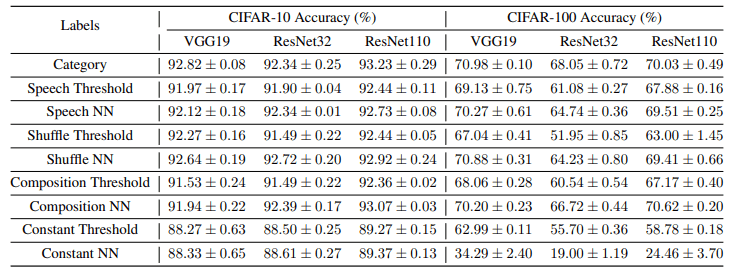Speech labels, shuffle speech labels, and mixed Gaussian labels show comparable performance to conventional categorization labels, while constant matrix labels show slight performance degradation.

The learning curve is shown in the following figure, which also shows the difficulty of training using constant matrix labels.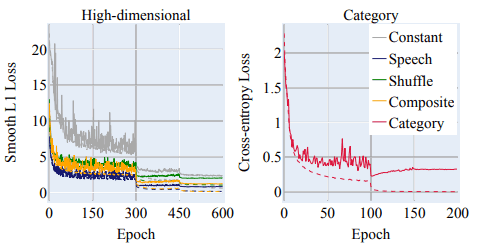To evaluate the robustness of the model, we perform an Adversarial Attack using FGSM and an iterative method. In this case, we perform the attack only on correctly classified images to investigate how well the performance is maintained.

The results (test accuracy) are shown in the figure below.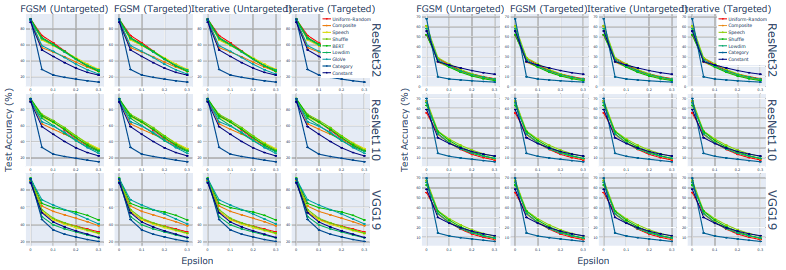The left side of the figure shows the results of the runs on CIFAR-10 and the right side shows the results of the runs on CIFAR-100. All model accuracies decrease with attack strength (abscissa: Epsilon), but the speech/shuffle speech/Gaussian labels perform significantly better than the traditional category labels in all cases.

The uniform-random matrices (Uniform-Random/Lowdim) showed excellent performance even in the low-dimensional case, while the labels consisting of constant matrices (Constant: purple line) showed relatively low performance. This suggests that some property other than the high dimensionality of the labels improves the robustness of the model.

### On training data efficiency

For the CIFAR-10 data set, we train using only 1%, 2%, 4%, 8%, 10%, and 20% of the training data. The test accuracy, in this case, is as follows.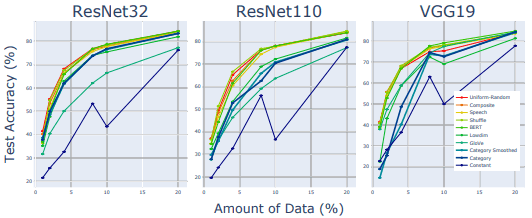Overall, the results are similar to those of the robustness evaluation. In particular, the performance of the constant matrix label is lower than that of the other high-dimensional labels, suggesting that some characteristics other than high dimensionality affect the performance.

However, the results for low-dimensional uniform random matrices (Lowdim) differ from the robustness assessment and show inferior results compared to high-dimensional matrices.

### Effectiveness of high-dimensional and high-entropy representations

The paper hypothesizes "high-dimensional label representation with high entropy" as a property of label representation that is useful for effective feature representation, which improves label robustness and data efficiency.

For this hypothesis, we present the statistics for the various label representations in the following table.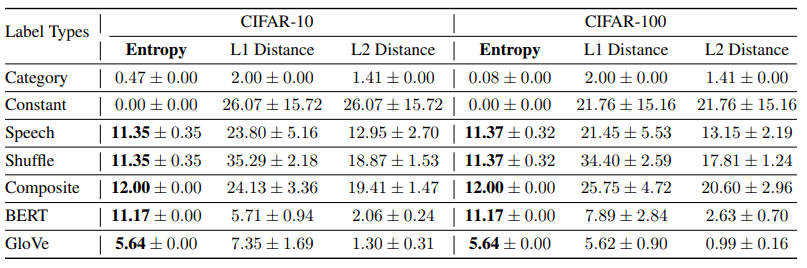L1/L2 Distance shows the distance between different label pairs.

The entropy of the labels that show the best results in terms of robustness and data efficiency (e.g., speech/shuffle speech/mixed Gaussian) are all higher than those of the regular category labels and constant matrix labels, reinforcing the previous hypothesis.

### Feature Visualization

The results of feature visualization using t-SNE for the case of ResNet-110 trained on CIFAR-10 are as follows.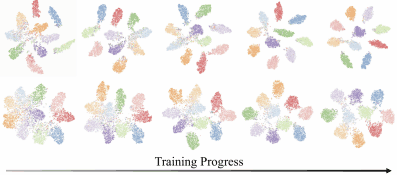The top row shows the results when using speech labels, and the bottom row shows the results when using category labels. Learning progresses from left to right, and 10%, 30%, 50%, 70%, and 100% of learning is completed, respectively.

Compared to category labels, the feature representation of speech labels showed that clusters were formed in the early stages of training and became more separated as training progressed.

## Summary

In the paper presented in this article, we showed that replacing category labels in image classification tasks with high-dimensional and high-entropy matrices such as audio spectrograms provides excellent robustness and data efficiency.

The fact that we have shown that label representations affect model performance leads to the question of whether the category labels used to train the model are really effective. This is an important study that provides a new perspective on the role of label representations.

If you have any suggestions for improvement of the content of the article,